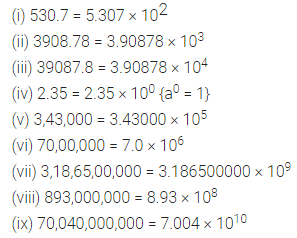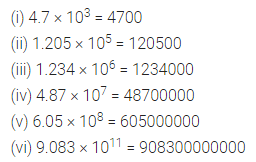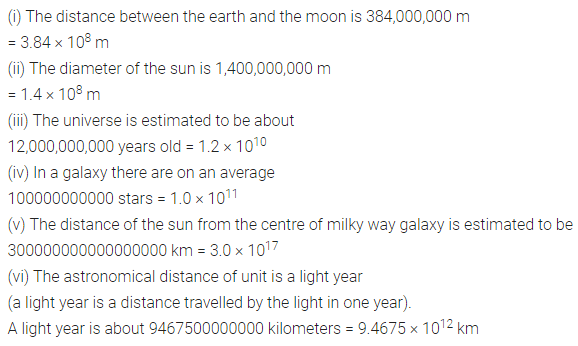# ML Aggarwal Class 7 Solutions for ICSE Maths Chapter 4 Exponents and Powers Ex 4.3

## ML Aggarwal Class 7 Solutions for ICSE Maths Chapter 4 Exponents and Powers Ex 4.3

Question 1.
Write the following numbers in the standard form (or scientific notation):
(i) 530.7
(ii) 3908.78
(iii) 39087.8
(iv) 2.35
(v) 3,43,000
(vi) 70,00,000
(vii) 3,18,65,00,000
(viii) 893,000,000
(ix) 70,040,000,000
Solution:Question 2.
Write the following numbers in usual decimal notation:
(i) 4.7 × 103
(ii) 1.205 × 105
(iii) 1.234 × 106
(iv) 4.87 × 107
(v) 6.05 × 108
(vi) 9.083 × 1011
Solution:Question 3.
Express the numbers appearing in the following statements in scientific notation (or standard form):
(i) The distance between the earth and the moon is 384,000,000 m.
(ii) The diameter of the sun is 1,400,000,000m.
(iii) The universe is estimated to be about 12,0000,000 years old.
(iv) In a galaxy, there are on an average 100,000,000,000 stars.
(v) The distance of the sun from the centre of milky way galaxy is estimated to be 300,000,000,000,000,000 km.
(vi) The astronomical distance of unit is a light-year (a light-year is a distance travelled by the light in one year). A light year is about 9,467,500,000,000 kilometers.
Solution:Question 4.
Compare the following numbers:
(i) 4.3 × 1014; 3.01 × 1017
(ii) 1.439 × 1012; 1.4335 × 1012
Solution:Question 5.
Write the following numbers in the expanded Exponential form:
(i) 279404
(ii) 3006194
(iii) 28061906
Solution:Question 6.
Find the number from each of the expanded form:
(i) 3 × 104 + 7 × 102 + 5 × 100
(ii) 4 × 105 + 5 × 103 + 3 × 102 + 2 × 100
(iii) 8 × 107 + 3 × 104 + 7 × 103 + 5 × 102 + 8 × 101
Solution: